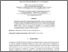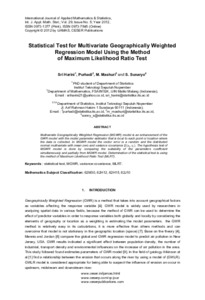# Statistical Test for Multivariate Geographically Weighted Regression Model Using the Method of Maximum Likelihood Ratio Test

Harini, Sri and Purhadi, Purhadi and Sunaryo, S (2012) Statistical Test for Multivariate Geographically Weighted Regression Model Using the Method of Maximum Likelihood Ratio Test. International Journal of Applied Mathematics and Statistics, 29 (5). pp. 110-115. ISSN 0973-1377 (Print), 0973-7545 (Online)Preview
Text
1284-1316-1-Harini.pdf

Full text available at: http://www.ceser.in/ceserp/index.php/ijamas

## Abstract

Multivariate Geographically Weighted Regression (MGWR) model is an enhancement of the GWR model with the model parameter estimator that is local to each point or location where the data is collected. In MGWR model the vector error is a random and the distributed normal multivariate with mean zero and variance covariance Σ(u_i,v_i ). The hypothesis test of MGWR model is done by comparing the suitability of the parameters coefficient simultaneously and partially from MGWR model. Determination of the statistical test is using the method of Maximum Likelihood Ratio Test (MLRT).

Item Type: Journal Article statistical test; MGWR; variance covariance; MLRT 01 MATHEMATICAL SCIENCES > 0104 Statistics > 010405 Statistical Theory Faculty of Mathematics and Sciences > Department of Mathematics Dr Sri Harini 27 Jun 2016 12:31View Item